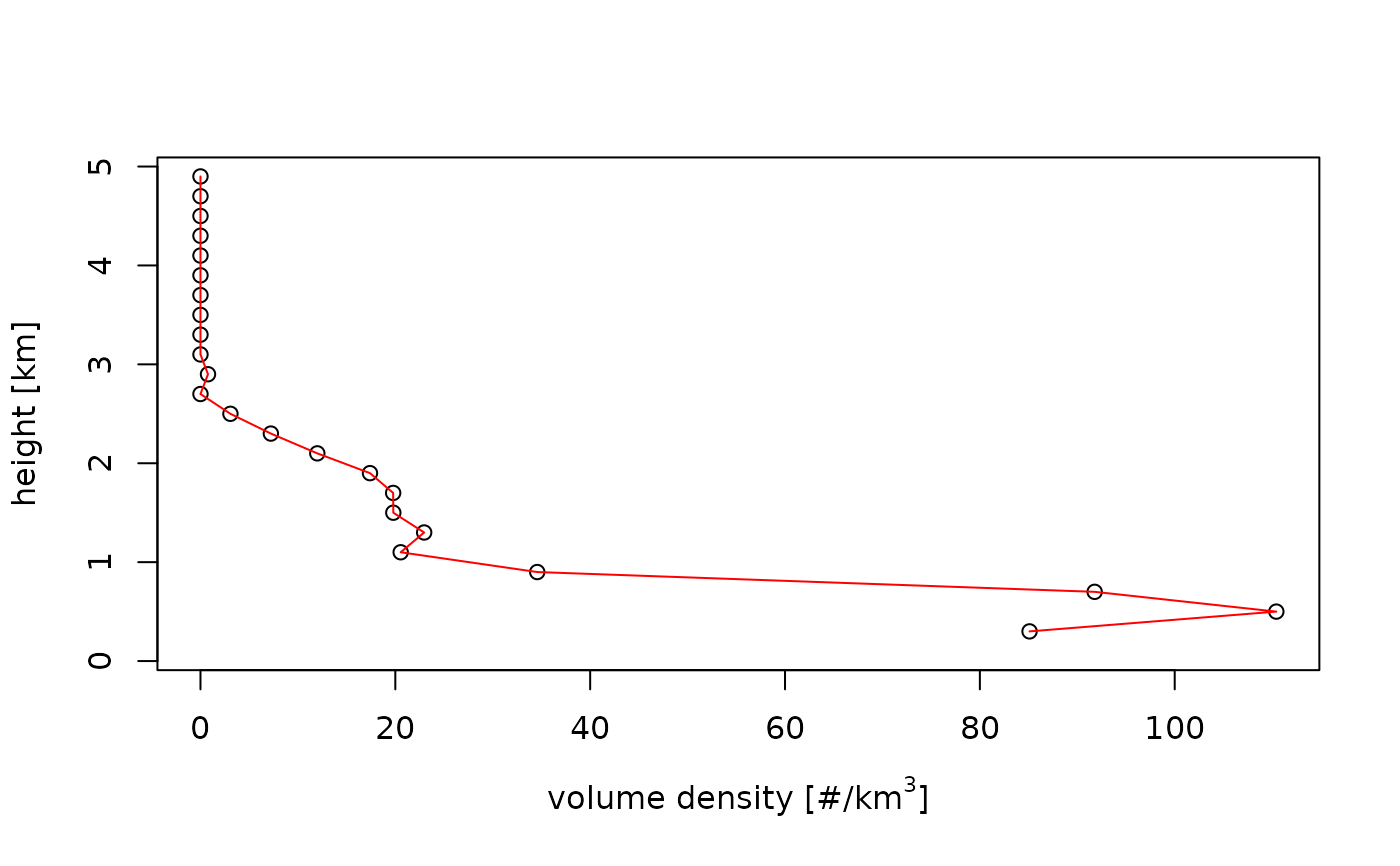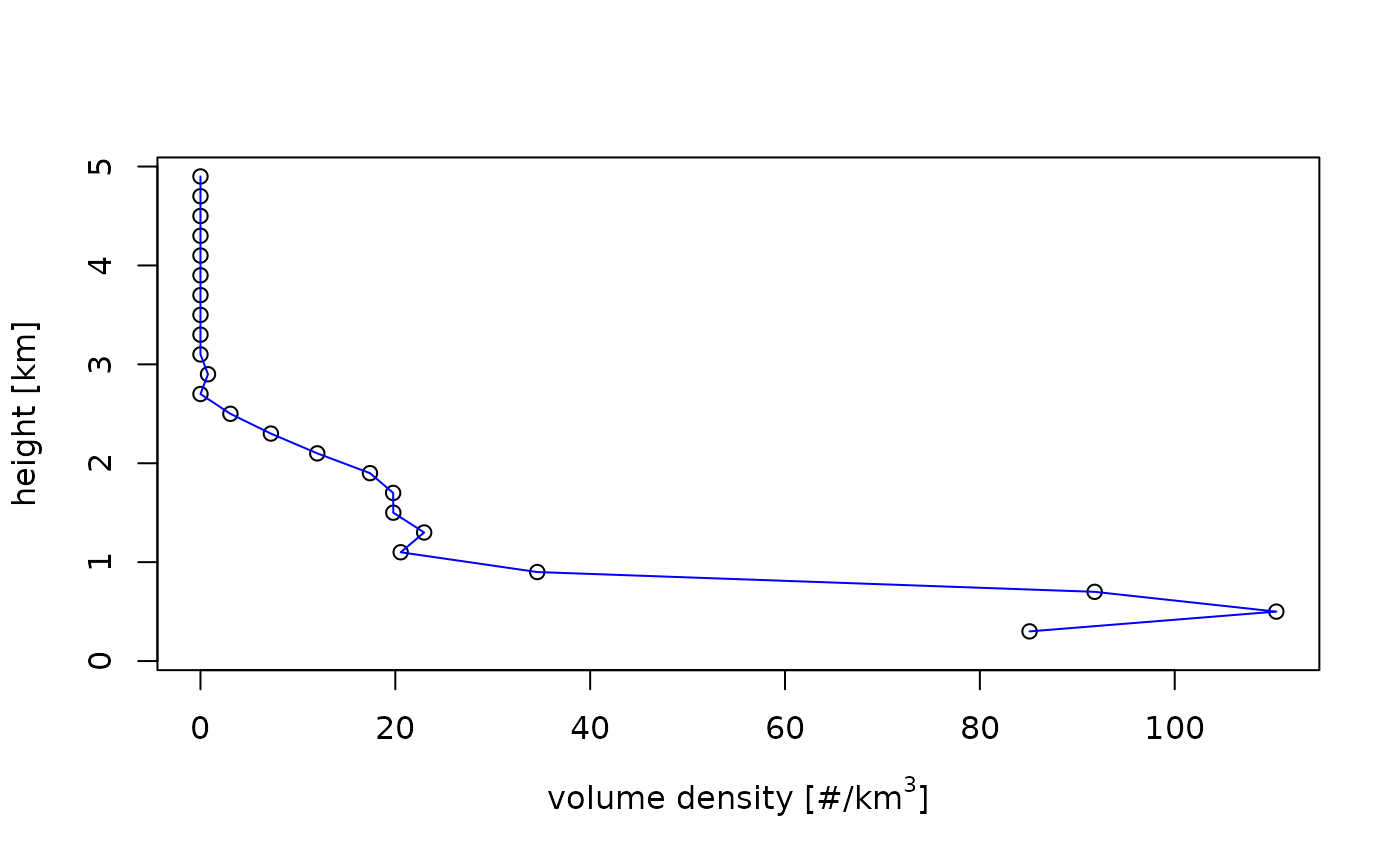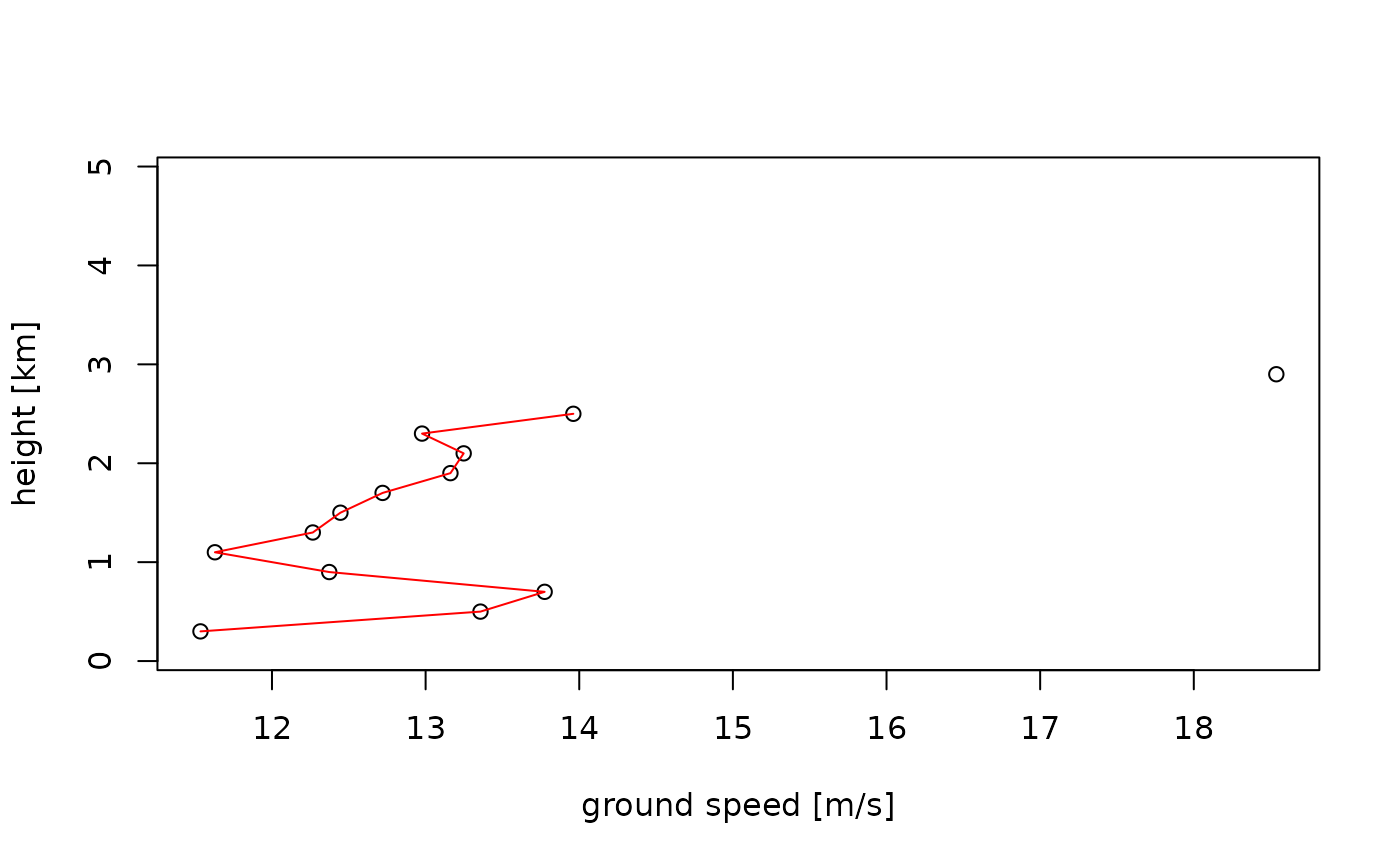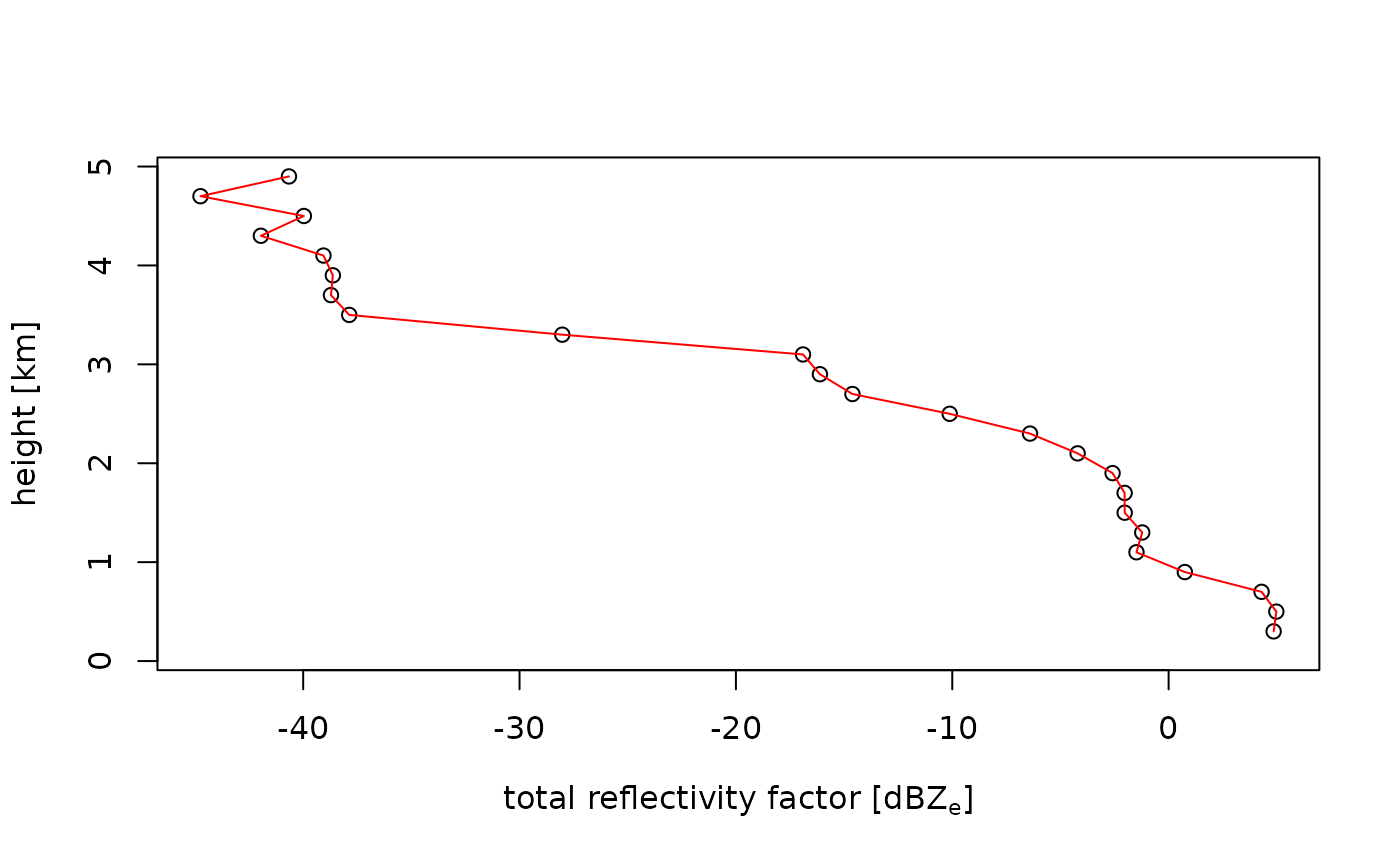Plot a vertical profile (vp)

# S3 method for vp
plot(
x,
quantity = "dens",
xlab = expression("volume density [#/km"^3 * "]"),
ylab = "height [km]",
line_col = "red",
line_lwd = 1,
line.col = "red",
line.lwd = 1,
...
)

## Arguments

x

A vp class object.

quantity

Character string with the quantity to plot. See vp for list of available quantities.

• Aerial density related:'dens', 'eta', 'dbz', 'DBZH' for density, reflectivity, reflectivity factor and total reflectivity factor, respectively.

• Ground speed related:'ff','dd', for ground speed and direction, respectively.

xlab

A title for the x axis.

ylab

A title for the y axis.

line_col

Color of the plotted curve.

line_lwd

Line width of the plotted curve.

line.col

line.lwd

...

Additional arguments to be passed to the low level plot plotting function.

## Examples

# load example vp object:
data(example_vp)

# plot the animal density:
plot(example_vp, quantity = "dens")# change the line color:
plot(example_vp, line_col = "blue")# plot the ground speed:
plot(example_vp, quantity = "ff")# plot the reflectivity factor of
# all scatterers (including precipitation):
plot(example_vp, quantity = "DBZH")